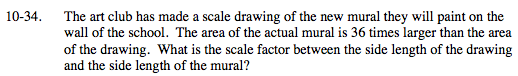### Home > MC2 > Chapter 10 > Lesson 10.1.3 > Problem10-34

10-34.Recall that area is always written in units2 while side lengths are always written in units.
This means that the ratio of of the two areas is 36 units2.
To find the ratio of the sides, find the square root of the ratio of the two areas.

This is the ratio of the side lengths of the larger mural to that of the scale drawing. The scale factor is therefore 6.

$\sqrt{\text{36 units^2}} = 6\text{ units}$